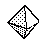Email us to get an instant 20% discount on highly effective K-12 Math & English kwizNET Programs!

#### Online Quiz (WorksheetABCD)

Questions Per Quiz = 2 4 6 8 10

### Grade 3 - Mathematics8.19 Geometry Review Test

 Q 1: What is the name of the shape of a football?Answer: Q 2: What do you call an angle that forms a square corner?obtuse angleright anglesquare angle Q 3: A rectangle has how many angles?324 Q 4: A regular polygon hasangles of equal measuresides of equal length and angles of equal measuresides of equal length Q 5: Perimeter of a square =4 x lengthlength + length2 x length Q 6: What is the shape with 8 faces, each formed by an equilateral triangle?Answer: Question 7: This question is available to subscribers only! Question 8: This question is available to subscribers only!

#### Subscription to kwizNET Learning System offers the following benefits:

• Unrestricted access to grade appropriate lessons, quizzes, & printable worksheets
• Instant scoring of online quizzes
• Progress tracking and award certificates to keep your student motivated
• Unlimited practice with auto-generated 'WIZ MATH' quizzes